Algebra 1 5-6 Complete Lesson: Parallel and Perpendicular Lines
starstarstarstarstarstarstarstarstarstar
3 (2 ratings)
by Matthew Richardson
| 26 Questions
Note from the author:
A complete formative lesson with embedded slideshow, mini lecture screencasts, checks for understanding, practice items, mixed review, and reflection. I create these assignments to supplement each lesson of Pearson's Common Core Edition Algebra 1, Algebra 2, and Geometry courses. See also mathquest.net and twitter.com/mathquestEDU.The outlined content above was added from outside of Formative.1
2
1
5 pts
Solve It! If you copied the graph, could you draw a new line that would not intersect the other lines in the graph?
Yes
No
2
10 pts
Solve It! Can you draw a line that will intersect one of the lines in such a way that the intersection forms four congruent angles? Explain.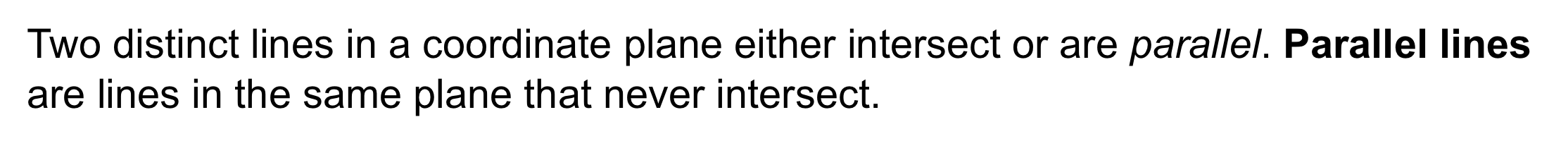3
3
10 pts
Problem 1 Got It?
A
B
C
D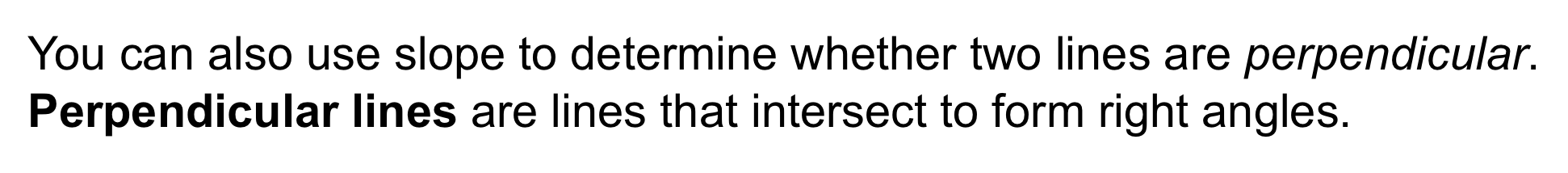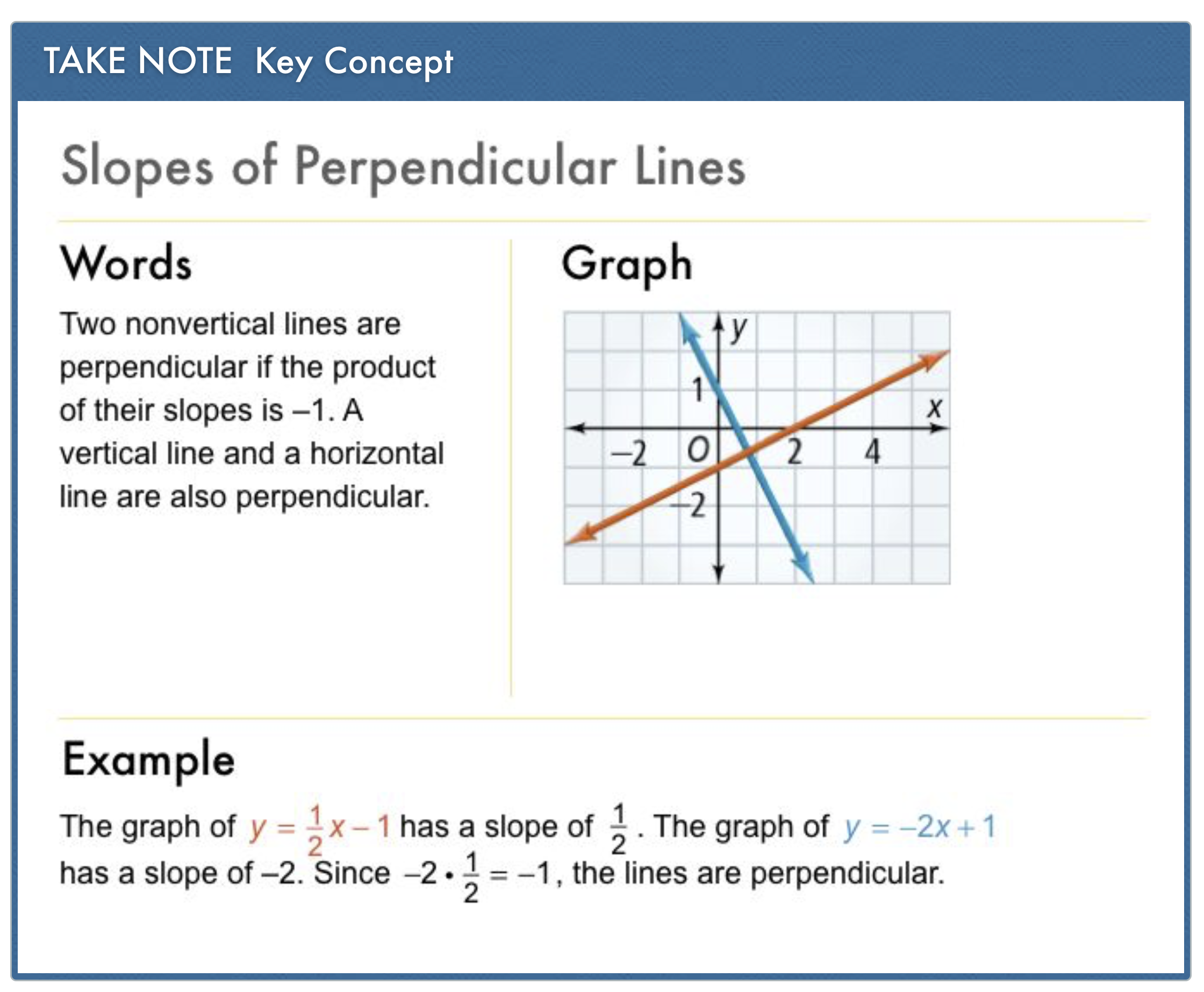4
4
10 pts
Problem 2 Got It?
A
B
C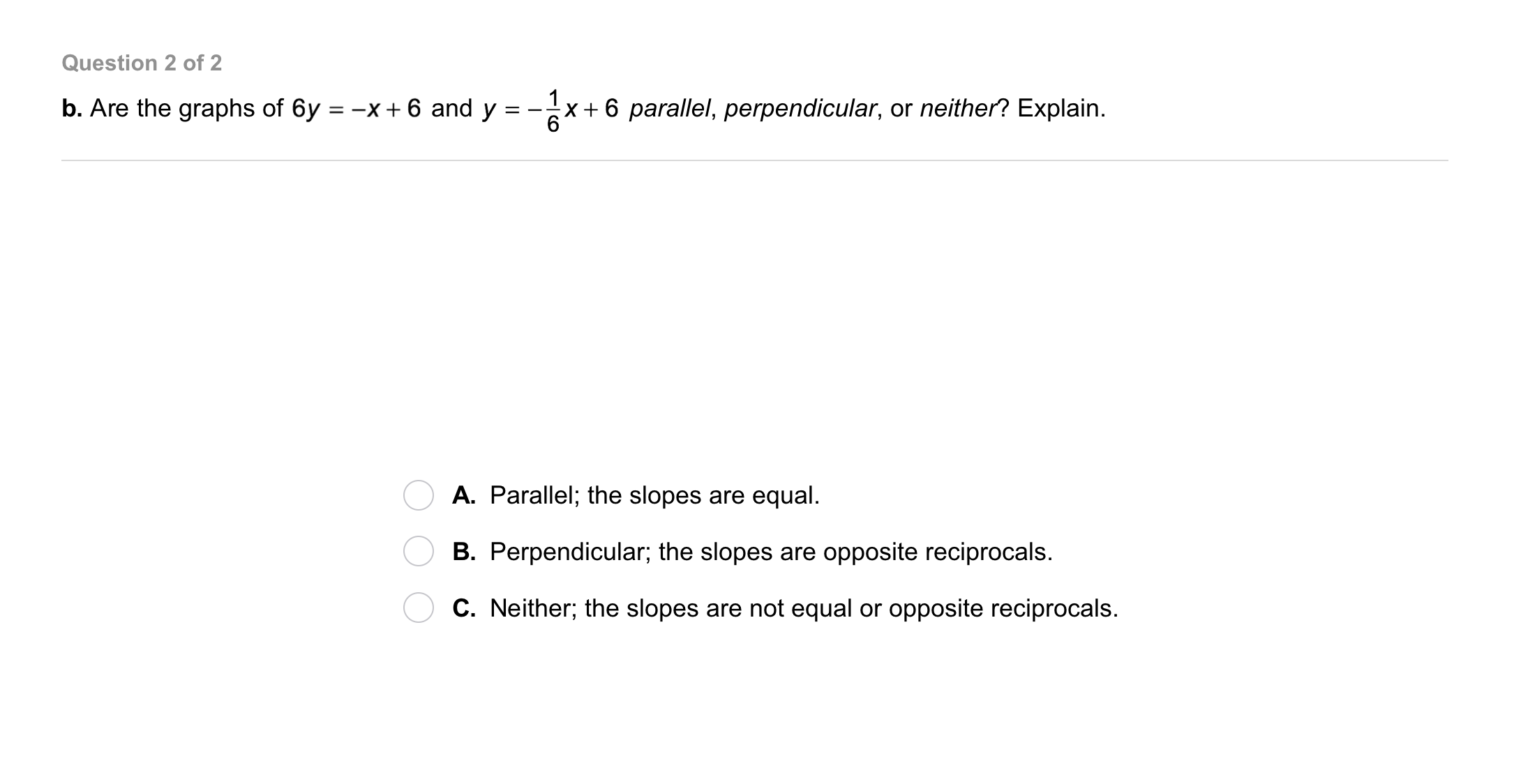5
5
10 pts
Problem 2 Got It?
A
B
C6
6
10 pts
Problem 3 Got It?
A
B
C
D7
7
10 pts
Problem 4 Got It? What equation could the architect enter to represent a second beam whose graph will pass through the corner at (0, 10) and be parallel to the existing beam. An equation that represents the existing beam is:

A
B
C
D8
10 pts
Which equation below has a graph that is parallel to the graph of the equation given?

y = 6x
y = 6x - 2
Both of the equations above
None of these
9
10 pts
Which equation below has a graph that is perpendicular to the graph of the equation given?

y = 6x
y = 6x - 2
Both of the equations above
None of these
10
10 pts
Which equation below has a graph that is parallel to the graph of the equation given?

y = 6x
y = 6x - 2
Both of the equations above
None of these
11
10 pts
What is an equation of the line that passes through (3, -1) and is parallel to the equation below?
y = 4x + 11
y = -4x + 11
y = (1/4)x + 11
y = (-1/4)x + 11
12
10 pts
What is an equation of the line that passes through (2, -3) and is perpendicular to y = x - 5?

y = x + 1
y = -x + 1
y = -x - 1
y = x - 1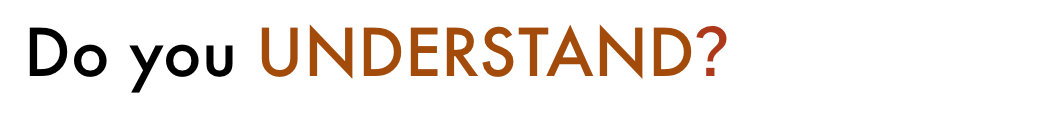13
5 pts
Vocabulary: The two numbers in the pair are opposite reciprocals.

True
False
14
5 pts
Vocabulary: The two numbers in the pair are opposite reciprocals.

True
False
15
5 pts
Vocabulary: The two numbers in the pair are opposite reciprocals.

True
False
16
10 pts
Open-Ended: Write equations of two parallel lines.
17
10 pts
Compare and Contrast: How is determining if two lines are parallel similar to determining if they are perpendicular? How are the processes different?18
10 pts
Review Lesson 5-5: What is the x-intercept of the line given by the equation?

(4, 4)
(7, 1)
(8, 0)
19
10 pts
Review Lesson 5-5: What is the y-intercept of the line given by the equation?

(4, 4)
(2, 6)
(0, 8)
(8, 0)
20
10 pts
Review Lesson 5-5: Graph the equation using x- and y-intercepts. Identify the intercepts clearly.
Be sure to include relevant graph detail: label axes, indicate units on both axes, and use arrows to represent end behavior, as appropriate.
21
10 pts
Review Lesson 5-5: Graph the equation using x- and y-intercepts. Identify the intercepts clearly.
Be sure to include relevant graph detail: label axes, indicate units on both axes, and use arrows to represent end behavior, as appropriate.
22
10 pts
Review Lesson 5-3: Write an equation in slope-intercept form of the line that passes through the given points.23
10 pts
Vocabulary Review: What is the product of a number and its reciprocal ?
1
100
-1
024
10 pts
Use Your Vocabulary: Complete each sentence with parallel or perpendicular.

• parallel
• perpendicular
• The railroad tracks in Picture A are __?__.
• The window bars in Picture B that do NOT meet are __?__.
• The roads in Picture C are __?__.
25
100 pts
Notes: Take a clear picture or screenshot of your Cornell notes for this lesson. Upload it to the canvas. Zoom and pan as needed.

For a refresher on the Cornell note-taking system, click here.
26
10 pts
Reflection: Math Success
Add to my formatives list

Formative uses cookies to allow us to better understand how the site is used. By continuing to use this site, you consent to the Terms of Service and Privacy Policy.